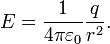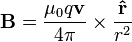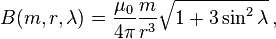# Electric fields - magnetic fields?

kashiark
If an electric field causes 2 previously stationary charges to accelerate(let's say that there was something inhibiting their movement that has now been removed) toward or away from one another, what type of field is between them, electric or magnetic?

## Answers and Replies

Gold Member
Accelerating charges produce electromagnetic radiation. You would expect to find both electric and magnetic fields.

kashiark
So how would you calculate the force at any point in time assuming you knew the distance at that particular point?

Gold Member
Using the Lorentz Force:

$$\mathbf{F} = q\left(\mathbf{E} + \mathbf{v}\times\mathbf{B}\right)$$

kashiark
Ok, let's say we have a positron and an electron moving toward one another; which way would the magnetic field point? Does it depend on their spins? If so, what if they have the same spin?

kashiark
Isn't the magnetic moment of an electron (and positron for that matter) dependent on the spin? And isn't the magnetic field dependent on the magnetic moment?

Gold Member
The magnetic moment due to the spin is negligible for what you are talking about.

varga
If an electric field causes 2 previously stationary charges to accelerate(let's say that there was something inhibiting their movement that has now been removed) toward or away from one another, what type of field is between them, electric or magnetic?

Rather than "between" I'll say 'around' each moving electric charge there will be one electric field and three magnetic fields.

1x Electric field, described with Coulomb's law:1x Magnetic field 'due to moving charge', described with Biot-Savart law:2x Magnetic fields 'due to spinning charge', described with magnetic dipole equation:However, the magnetic field 'due to moving charge', the one most important here, in the case when electrons are orbiting nucleus will describe doughnut shaped magnetic field whose superposition through time will actually form a dipole moment, so in total there would be four magnetic fields and one electric field around an electron circling a proton. This 'doughnut' or "compound magnetic field" due to electron circular orbit around nucleus will create two opposite magnetic fields, above and below the ring. This dipole moment is what is responsible for magnetism in permanent magnets, not the magnetic dipole moment due to electron spin, or so they say.

Ok, let's say we have a positron and an electron moving toward one another; which way would the magnetic field point? Does it depend on their spins? If so, what if they have the same spin?

Use Lorentz force and Biot-Savart law to calculate their motion and the forces of attraction and repulsion to know which way will they move. Two parallel wires attract if they carry current in the same direction and they repel if the current goes in opposite direction.

So, considering only this magnetic field described by Biot-Savart law, if two electrons passing each other in opposite directions repel, that means positron and electron moving toward one another will ATTRACT.

However, if you further model this interaction you will find they will attract and repel depending on their position, velocity and relative orientation, in other words they will continue oscillate around each other, if they come close enough. You could say they will form an electric dipole which due to superposition principle might appear as having zero electric charge... these two particles interacting together would look a lot like a photon or em radiation.

Actually, in the process called 'Pair_production' photons will "split" in electron-positron pairs and in the opposite process called 'Positron-electron_annihilation', these two types of particles form photons or em radiation.

Isn't the magnetic moment of an electron (and positron for that matter) dependent on the spin? And isn't the magnetic field dependent on the magnetic moment?

There is that, which is usually called "magnetic dipole moment due to intrinsic spin", and there is the one described with Biot-Savart law and is called "magnetic field due to moving charge". Later one apperantly have much greater influence and is responsible for magnetism in permanent magnets, the magnetic dipole moment due to spin kind of cancels itself out since it has two opposite fields close together, or so it would seem.

Last edited:
kashiark
Thanks! You've cleared up many of my misconceptions, but I don't understand your last statement. "The magnetic dipole moment due to spin kind of cancels itself out since it has two opposite fields close together, or so it would seem." Do you mean when the electrons are in pairs or will just one electron exhibit this behavior?

varga
Any single electron has this "intrinsic spin" which indirectly means "magnetic dipole moment", which implies "two opposite magnetic fields". Now, it is actually according to classical physics that spinning charge would result in creation of two opposite magnetic fields, however these magnetic forces are fact of measurement and then it turned out electron "size" is too big (?!?) and so it would be rotating faster than the speed of light if this magnetic moment was to be due to the real rotation, so they decided electrons are not actually spinning but simply have this property ironically named "intrinsic spin", which by definition automatically comes with these two magnetic fields, just like that.

There is another theory that says electrons do not have size and so the very meaning of "rotation" looses its logic since there is no radius and there is no point on its surface that could actually be moving faster than the speed of light, or at all.

Anyhow, the closer opposite fields are, the better they neutralize each other, but this magnetic dipole moment due to spin has constant values according to measurements, I just wanted to say the range of this particular dipole moment is diminished in comparison to the magnetic field described by the Biot-Savart law, as the magnitude of those fields drops quickly with the inverse cube law.

Last edited:
kashiark
So if an electron were stationary in space, it would have an extremely weak north side and a south pole on two sides opposite one another?

varga
Yes, they are diametrically opposite, just like those two field above and below the ring electron makes while circling proton. Actually if you take the point on electron surface, then spin it, the mechanics of it would be the same as if electron itself is made of similar electric loop. -- I would not say "weak" because the actual forces are something that depends on the distance and even seemingly weak things can be pretty strong if you get close enough. I would not call the fields "north and south" because it's relation of directions, not absolute.

Whether stationary or not, magnetic dipole moment of an electron is supposed to be of the same magnitude, always. I'm not sure how strong that is, but it seem much less than the one due to spatial velocity (Biot-Savart) even with relatively slow velocities. But the important thing is its RANGE diminishes quickly with the distance because it drops of with the inverse cube law, see magnetic dipole equation above.

It is the 'magnetic field of a moving charge' that is proportional to velocity. Not only this magnetic field can get very "strong" as the velocity increases, but it's range drops only with the inverse square law, see Biot-Savart law above.

If there is such thing as stationary electron it would have only two magnetic fields from this "intrinsic spin" magnetic dipole moment, the field described by Biot-Savart law would not exist as zero velocity would mean zero magnetic potential of this particular field.

kashiark
Ok, one more question and then I'm done.
F = q(E + v cross B)
Can someone do one of these as an example? Thanks!

Gold Member
Ok, one more question and then I'm done.
F = q(E + v cross B)
Can someone do one of these as an example? Thanks!

I don't quite understand. Are you familiar with vector mathematics? This is a fairly straightforward equation. E and B are the electric and magnetic field vectors respectively, q is the charge of your charged particle, and v is the velocity vector of your charge.

kashiark
Nevermind; I had a problem, but I figured it out. Thanks everyone!